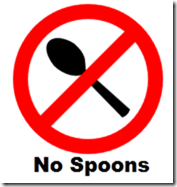And now since I'm in the mood for writing some XAML, here's something really silly. My 2 year old son always throws his spoon in the bin along with his empty yoghurt pot, which has meant we are regularly buying more spoons. So here is a really useful XAML "No Spoons" sign for the bin. I 'designed' the spoon icon in Blend, and then used a transform group to get it into the right place.

Here's the code:

``````<Canvas xmlns="http://schemas.microsoft.com/winfx/2006/xaml/presentation">
<Canvas Canvas.Left="20" Canvas.Top="20">
<Canvas>
<Path Fill="#FF000000" Stroke="#FF000000" Data="M438.66803,130.33333 C421.33499,120.1762 422.00165,96.176278 422.00165,96.176278 427.00179,58.843133 452.66745,63.333794 460.00086,94.666778 461.00102,116.50951 455.41396,118.92027 446.00105,130.00013 L450.41981,208.7423 C446.16375,210.81869 441.91328,210.84422 437.66793,209 z" StrokeThickness="2"/>
<Canvas.RenderTransform>
<TransformGroup>
<TranslateTransform X="-350" Y="-60" />
<RotateTransform Angle="-45" CenterX="100" CenterY="100" />
<ScaleTransform ScaleX="0.75" ScaleY="0.75" />
<TranslateTransform X="25" Y="15" />
</TransformGroup>
</Canvas.RenderTransform>
</Canvas>
<Canvas Canvas.Left="10" Canvas.Top="10">
<Ellipse Height="150" Width="150" Stroke="Red" StrokeThickness="15" />
<Line StrokeThickness="15" Stroke="Red" X1="25" Y1="125" X2="125" Y2="25" />
</Canvas>
<TextBlock Canvas.Top="160" Canvas.Left="30" Text="No Spoons" FontFamily="Arial Black" Foreground="Black" FontSize="20"  />
</Canvas>
</Canvas>
``````

And here's what it looks like: